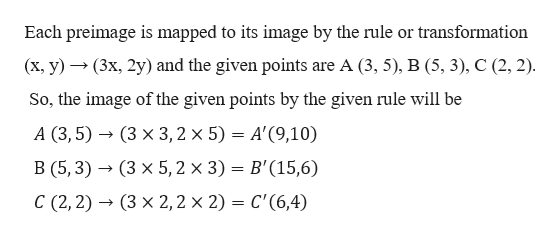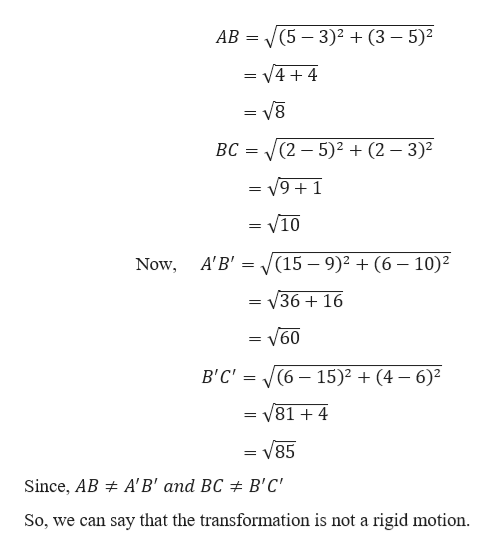Use the rule (x, y) → (3x, 2y) to find the image for the preimage defined by thepoints. Determine whether the transformation is a rigid motion. 4. A(3, 5) , B(5, 3) , C(2, 2)How would I be able to work this step by step?

Question

Use the rule (x, y) → (3x, 2y) to find the image for the preimage defined by the
points. Determine whether the transformation is a rigid motion.
4. A(3, 5) , B(5, 3) , C(2, 2)

How would I be able to work this step by step?

Step 1

Steps to solve this problem

• 1st find the image of the preimage of the points by the given transformation.
• Measure the distance between the images.
• Measure the distance between the preimages.
• If the distance between the images is equal to the distance between the corresponding preimages, then the transformation is rigid motion otherwise it is not a rigid motion
Step 2

Given,help_outlineImage TranscriptioncloseEach preimage is mapped to its image by the rule or transformation (x, y)(3x, 2y) and the given points are A (3, 5), B (5, 3), C (2, 2) So, the image of the given points by the given rule will be A (3, 5)(3 x 3, 2 x 5) = A'(9,10) B (5,3)(3 x 5, 2 x 3) B'(15,6) C (2, 2)(3 x 2,2 x 2) = C'(6,4) fullscreen
Step 3

Calculati...help_outlineImage TranscriptioncloseАВ -D (5 — 3)? + (3 — 5)? = V4 4 BC (2-5)2 (2 3)2 = V9 + 1 V10 A'B' = (15-9)2 (6 10)2 Now = V36 + 16 = V60 (6 15)2(4 - 6)2 В'С" V81 + 4 V85 Since, AB A'B' and BC B'C' So, we can say that the transformation is not a rigid motion fullscreen

Want to see the full answer?

See Solution

Want to see this answer and more?

Our solutions are written by experts, many with advanced degrees, and available 24/7

See Solution
Tagged in

Coordinate Geometry